# GRE Subject Test: Chemistry : Reaction Rates and Reaction Order

## Example Questions

### Example Question #1 : Reaction Rates And Reaction Order

A scientist is studying a reaction, and places the reactants in a beaker at room temperature. The reaction progresses, and she analyzes the products via NMR. Based on the NMR readout, she determines the reaction proceeds as follows: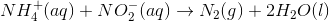In an attempt to better understand the reaction process, she varies the concentrations of the reactants and studies how the rate of the reaction changes. The table below shows the reaction concentrations as she makes modifications in three experimental trials.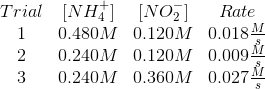Reaction rates depend on reaction concentrations, as well as the reaction constant. The Arrhenius equation is used to calculate this constant. Which of the following factors is used in the Arrhenius equation?

I. Temperature

II. Activation energy

III. Reactant concentrations

I only

II and III

I, II, and III

I and II

III only

I and II

Explanation:

If you thought all of these factors are used in the Arrhenius equation, consider that the rate constant is independent of the reactant concentrations. The concentration effect is considered in the rate law by multiplying the concentrations by the constant, but the concentrations themselves are independent of the actual Arrhenius calculation.

The calculation shows that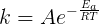. In this equation,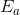is the activation energy and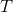is the temperature.

### Example Question #1 : Reaction Rate And Rate Law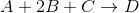You want to find the order of each reactant by manipulating the reactant concentrations in multiple trials. The table below shows the effect of altered reactant concentrations on initial reaction rate.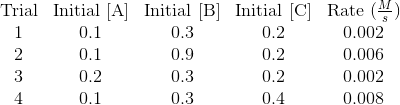Using the above trials, write the rate law for the reaction.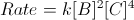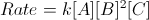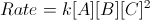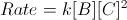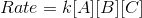Explanation:

Keep in mind that the chemical equation and its coefficients have NOTHING to do with the rate law. The order of each reactant must be determined by experiment.

To find the order of each reactant, compare the initial reaction rates of two trials in which only one of the three reactants' concentrations is altered. For example, trials 1 and 4 keep A and B equal, but C is doubled. When C is doubled, we see that the initial reaction rate is quadrupled. As a result, we determine that the order of reactant C is 2. When the reactant is altered, but the initial reaction rate is kept constant, as seen in trials 1 and 3 with respect to A, the order of that reactant is 0. Finally, when the reactant is multiplied by the same factor that the initial reaction rate is multiplied, as seen in trials 1 and 2 with respect to B, the order of the reactant is 1.

Putting the data together: A is zeroth order, B is first order, and C is second order. Our rate law can thus be written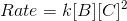.

### Example Question #11 : Reaction Rate And Rate Laws

A scientist is studying a reaction, and places the reactants in a beaker at room temperature. The reaction progresses, and she analyzes the products via NMR. Based on the NMR readout, she determines the reaction proceeds as follows:In an attempt to better understand the reaction process, she varies the concentrations of the reactants and studies how the rate of the reaction changes. The table below shows the reaction concentrations as she makes modifications in three experimental trials.Which of the following most closely approximates the rate law for this reaction?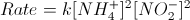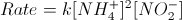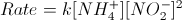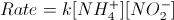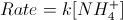Explanation:

The reaction table in the passage indicates that the reaction rate varies in a 1-to-1 fashion as you vary the each reactant, while holding the other constant.

The rate law is written as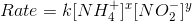.

Compare trials 1 and 2 to see that doubling the ammonium concentration doubles the rate. The reaction is first order for ammonium:.

Compare trials 2 and 3 to see that tripling nitrate concentration triples the rate. The reaction is first order for nitrate: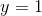.

The final rate law is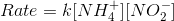.

### Example Question #1 : Reaction Rates And Reaction Order

For the reaction,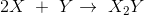, the initial rate of the reaction was determined and the values are tabulated below.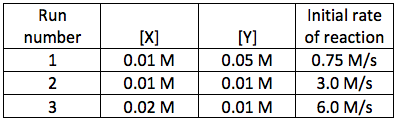What is the overall order for the rate law of this reaction?Explanation:

For the reaction given: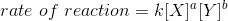We need to find the reaction order, therefore we have to find the values of a and b in the equation. First, let us compare the results of the experiments done to determine how changing the concentration effects the rate of the reaction. Let's compare run number 1 with run number 2 in which the concentration of Y was doubled in run 2 while keeping the concentration of X constant. We can observe that the reaction rate was quadrupled. Let's compare by ratios: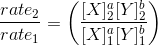The value for the concentration of B was the same for run 1 and 2 and therefore they cancel out: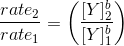Plugging the values into the equation gives: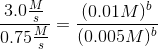Simplifying the above equation gives: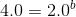Therefore,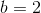We can also compare the results of run number 2 and run number 3. In that case, the concentration of Y was kept constant while the concentration of X was doubled. We can see that the reaction rate doubled. Therefore the reaction is first order with respect to X and second order with respect to Y. Therefore, the reaction is third order overall: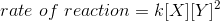### Example Question #1 : Kinetics And Kinematics

For the reaction,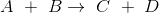, the initial rate of the reaction was determined and the values are tabulated below.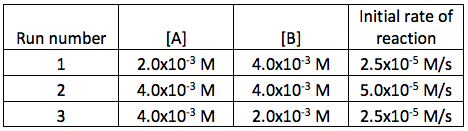Determine the overall order for the rate law of this reaction.Explanation:

For the reaction given: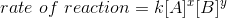We need to find the reaction order, therefore we have to find the values of x and y in the equation. First, let us compare the results of the experiments done to determine how changing the concentration effects the rate of the reaction. Let's compare run number 1 with run number 2 in which the concentration of A was doubled in run 2 while keeping the concentration of B constant. We can observe that the reaction rate was doubled. Let's compare by ratios: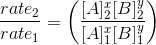The value for the concentration of B was the same for run 1 and 2 and therefore they cancel out: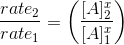Plugging the values into the equation gives: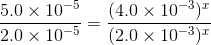Simplifying the above equation gives: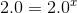Therefore,We can also compare the results of run number 2 and run number 3. In that case, the concentration of A was kept constant while the concentration of B was decreased by half. We can see that the reaction rate decreased by half. Therefore the reaction is first order with respect to A and first order with respect to B. Therefore, the reaction is second order overall: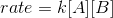### All GRE Subject Test: Chemistry Resources